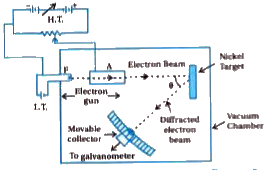Doubtnut App - Get 50 Lakh+ video solutions better than GoogleInstall Now

#Consider arrangement shown In figure for davisson-Germer experiment when voltage applied to A is increased in diffracted beam will have maximum number of electron for value diffraction angle (θ) such that…..Solution in Gujrati

A

Will be larger than the earlier value.

B

Will be the same as the earlier value

C

Will be less than the earlier value

D

Will depend on the target

Text Solution
Verified by Experts

## In the present case ,Path diffrence between matter waves diffracted from the onsecutive atomic layers of targer element,separated by perpendiular distance d is equal to 2dsinθ (where θ=angle of diffraction). Now ,as per the statement,for no.Of electrons getting diffracted at angle θ to become maximum,value of matter wavelength of moving electrons.λ should be such that it can satisfy the condition of constructive interference. Thus ,2din θλ ∴ 2din θ=n(h√2Vem) ⇒ As V increases, sinθ decreases and so θ will also decrease. Second method: In Dacisson-Germer experiment let voltage applied to andoe is V.De-Broglie wavelength assocaited wth electron, λ=12.27√VÅ.......(1) For electron diffracted at angle θ condition for central maximum λ−2dsinθ.......(2) From equation (1) if V increase then λ will decrease and from equation (2) in λ decrease then θ will also decrease. (For samll angle of diffraction sinθ≈θ).